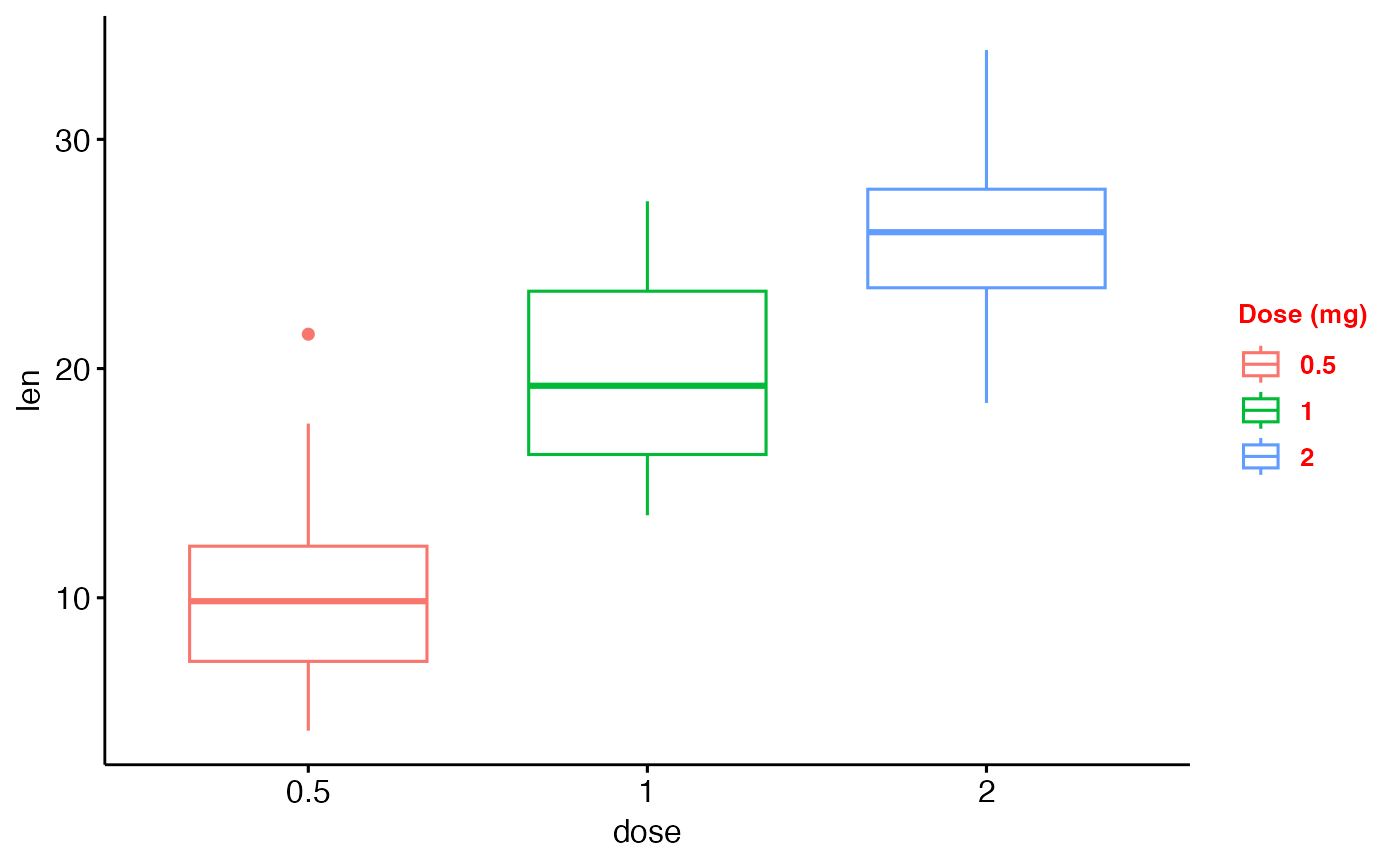Graphical parameters

ggpar(
p,
palette = NULL,
main = NULL,
submain = NULL,
caption = NULL,
xlab = NULL,
ylab = NULL,
title = NULL,
subtitle = NULL,
font.main = NULL,
font.submain = NULL,
font.x = NULL,
font.y = NULL,
font.caption = NULL,
font.title = NULL,
font.subtitle = NULL,
font.family = "",
xlim = NULL,
ylim = NULL,
xscale = c("none", "log2", "log10", "sqrt"),
yscale = c("none", "log2", "log10", "sqrt"),
format.scale = FALSE,
legend = NULL,
legend.title = NULL,
font.legend = NULL,
ticks = TRUE,
tickslab = TRUE,
font.tickslab = NULL,
font.xtickslab = font.tickslab,
font.ytickslab = font.tickslab,
x.text.angle = NULL,
y.text.angle = NULL,
xtickslab.rt = x.text.angle,
ytickslab.rt = y.text.angle,
xticks.by = NULL,
yticks.by = NULL,
rotate = FALSE,
orientation = c("vertical", "horizontal", "reverse"),
ggtheme = NULL,
...
)

## Arguments

p an object of class ggplot or a list of ggplots the color palette to be used for coloring or filling by groups. Allowed values include "grey" for grey color palettes; brewer palettes e.g. "RdBu", "Blues", ...; or custom color palette e.g. c("blue", "red"); and scientific journal palettes from ggsci R package, e.g.: "npg", "aaas", "lancet", "jco", "ucscgb", "uchicago", "simpsons" and "rickandmorty". Can be also a numeric vector of length(groups); in this case a basic color palette is created using the function palette. vector of colors to use for n-colour gradient. Allowed values include brewer and ggsci color palettes. plot main title. plot subtitle. plot caption. character vector specifying x axis labels. Use xlab = FALSE to hide xlab. character vector specifying y axis labels. Use ylab = FALSE to hide ylab. a vector of length 3 indicating respectively the size (e.g.: 14), the style (e.g.: "plain", "bold", "italic", "bold.italic") and the color (e.g.: "red") of main title, subtitle, caption, xlab and ylab, respectively. For example font.x = c(14, "bold", "red"). Use font.x = 14, to change only font size; or use font.x = "bold", to change only font face. alias of font.submain and font.submain, respectively. character vector specifying font family. a numeric vector of length 2, specifying x and y axis limits (minimum and maximum), respectively. e.g.: ylim = c(0, 50). x and y axis scale, respectively. Allowed values are one of c("none", "log2", "log10", "sqrt"); e.g.: yscale="log2". logical value. If TRUE, axis tick mark labels will be formatted when xscale or yscale = "log2" or "log10". character specifying legend position. Allowed values are one of c("top", "bottom", "left", "right", "none"). To remove the legend use legend = "none". Legend position can be also specified using a numeric vector c(x, y); see details section. legend title, e.g.: legend.title = "Species". Can be also a list, legend.title = list(color = "Species", linetype = "Species", shape = "Species"). legend text font style; e.g.: font.legend = c(10, "plain", "black"). logical value. Default is TRUE. If FALSE, hide axis tick marks. logical value. Default is TRUE. If FALSE, hide axis tick labels. Font style (size, face, color) for tick labels, e.g.: c(14, "bold", "red"). Numeric value specifying the rotation angle of x and y axis tick labels, respectively. Default value is NULL. For vertical x axis texts use x.text.angle = 90. Same as x.text.angle and y.text.angle, respectively. Will be deprecated in the near future. numeric value controlling x and y axis breaks, respectively. For example, if yticks.by = 5, a tick mark is shown on every 5. Default value is NULL. logical value. If TRUE, rotate the graph by setting the plot orientation to horizontal. change the orientation of the plot. Allowed values are one of c( "vertical", "horizontal", "reverse"). Partial match is allowed. function, ggplot2 theme name. Default value is theme_pubr(). Allowed values include ggplot2 official themes: theme_gray(), theme_bw(), theme_minimal(), theme_classic(), theme_void(), .... not used

## Examples

# Load data
data("ToothGrowth")
df <- ToothGrowth

# Basic box plot
# +++++++++++++++++++++++++++

p <- ggboxplot(df, x = "dose", y = "len")

# Change the plot orientation: horizontal
ggpar(p, orientation = "horiz")# Change main title and axis labels
# ++++++++++++++++++++++++++++

ggpar(p,
main = "Plot of length \n by dose",
xlab = "Dose (mg)", ylab = "Length")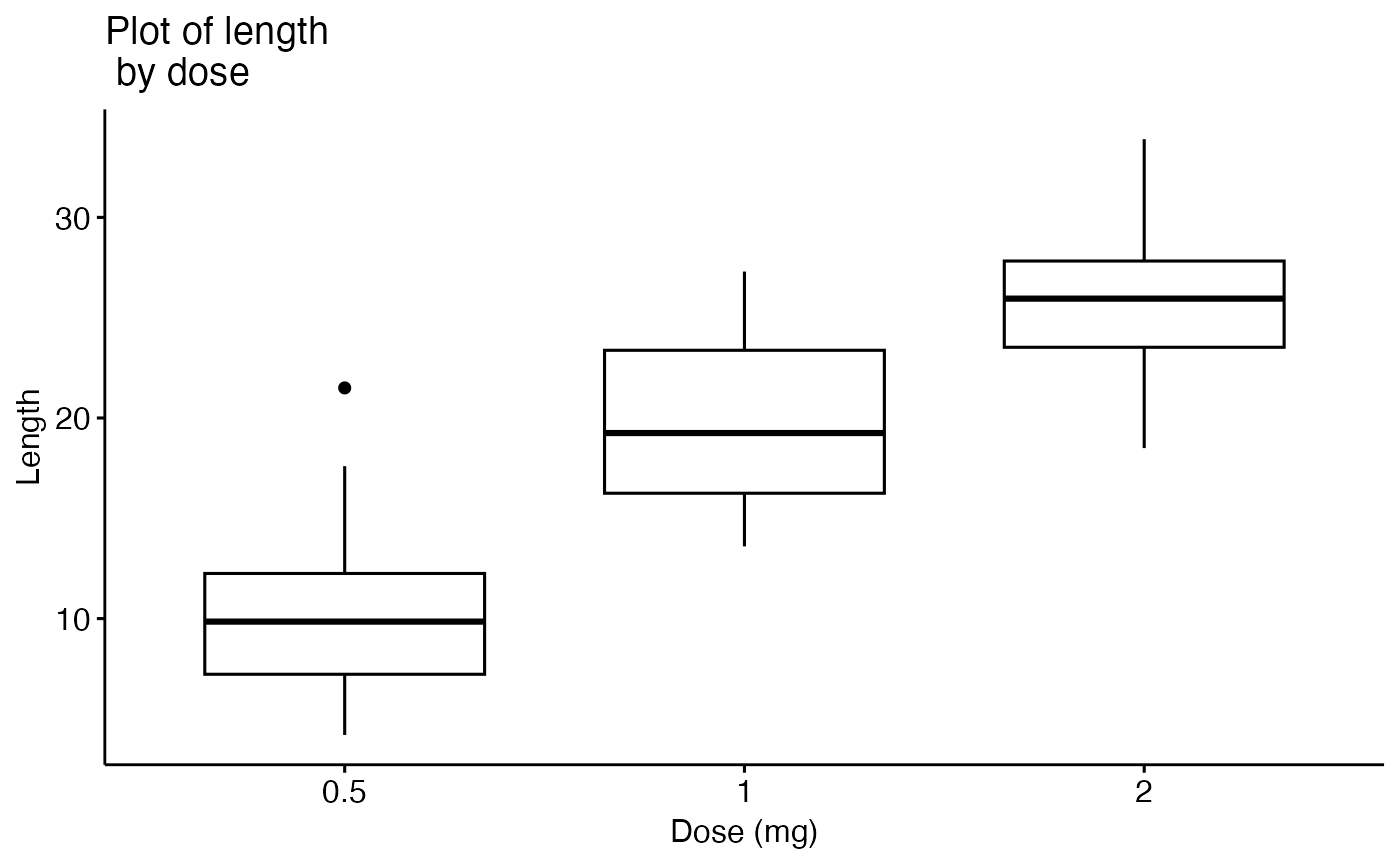# Title font styles: 'plain', 'italic', 'bold', 'bold.italic'
ggpar(p,
main = "Length by dose",
font.main = c(14,"bold.italic", "red"),
font.x = c(14, "bold", "#2E9FDF"),
font.y = c(14, "bold", "#E7B800"))# Hide axis labels
ggpar(p, xlab = FALSE, ylab = FALSE)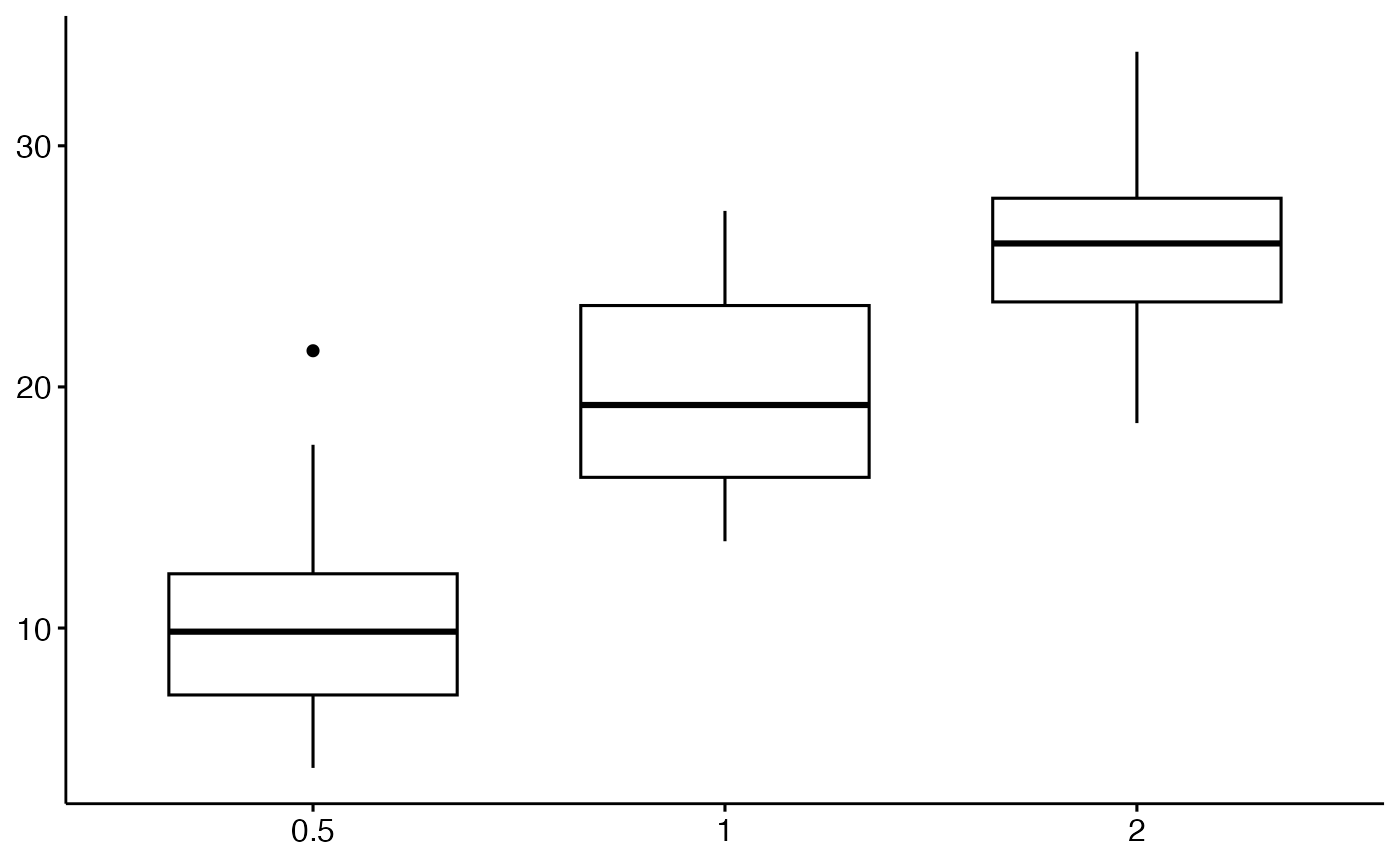# Change colors
# ++++++++++++++++++++++

# Change outline colors by groups: dose
p2 <- ggboxplot(df, "dose", "len", color = "dose")
p2# Use custom color palette
ggpar(p2, palette = c("#00AFBB", "#E7B800", "#FC4E07"))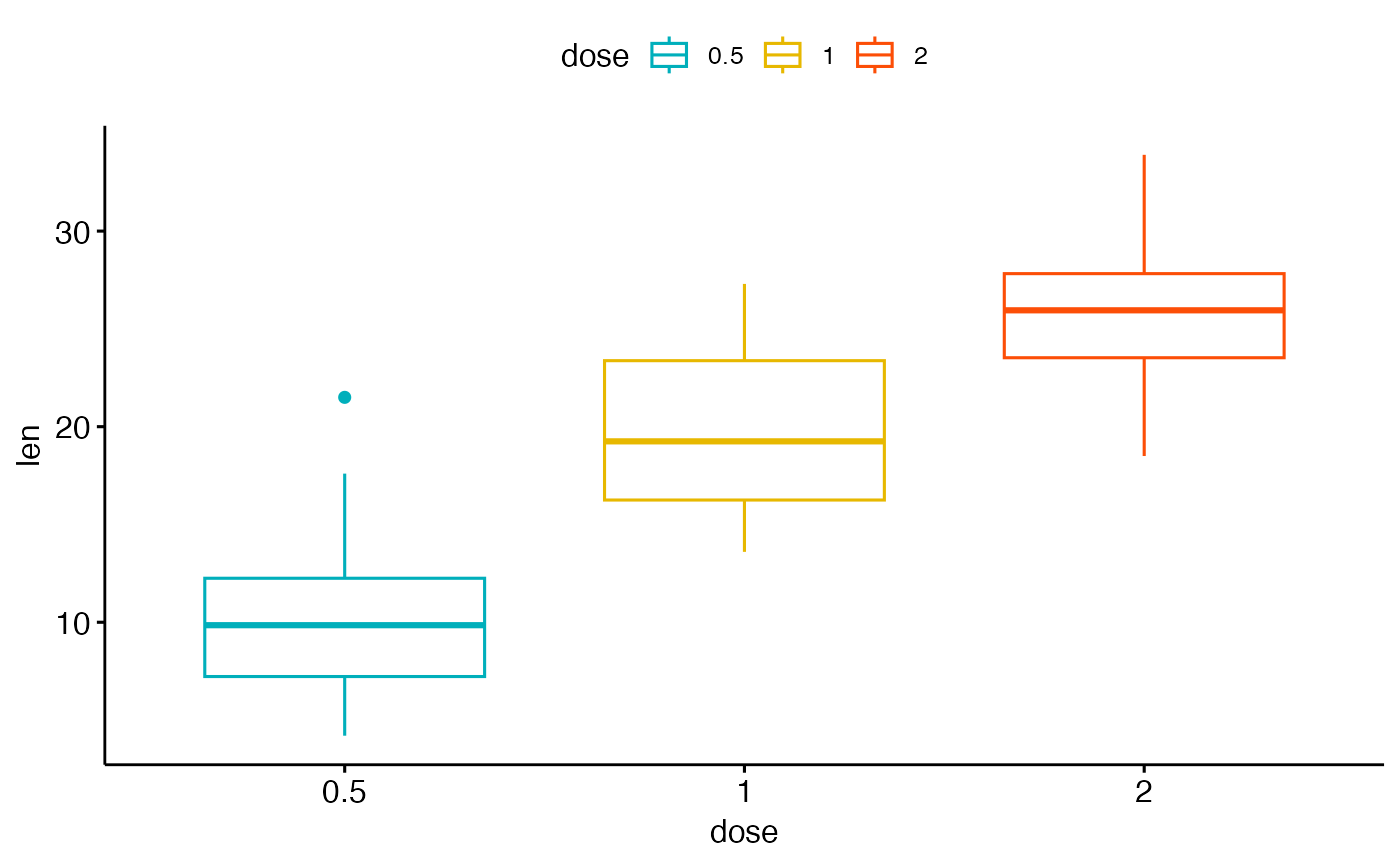# Use brewer palette
ggpar(p2, palette = "Dark2" )# Use grey palette
ggpar(p2, palette = "grey")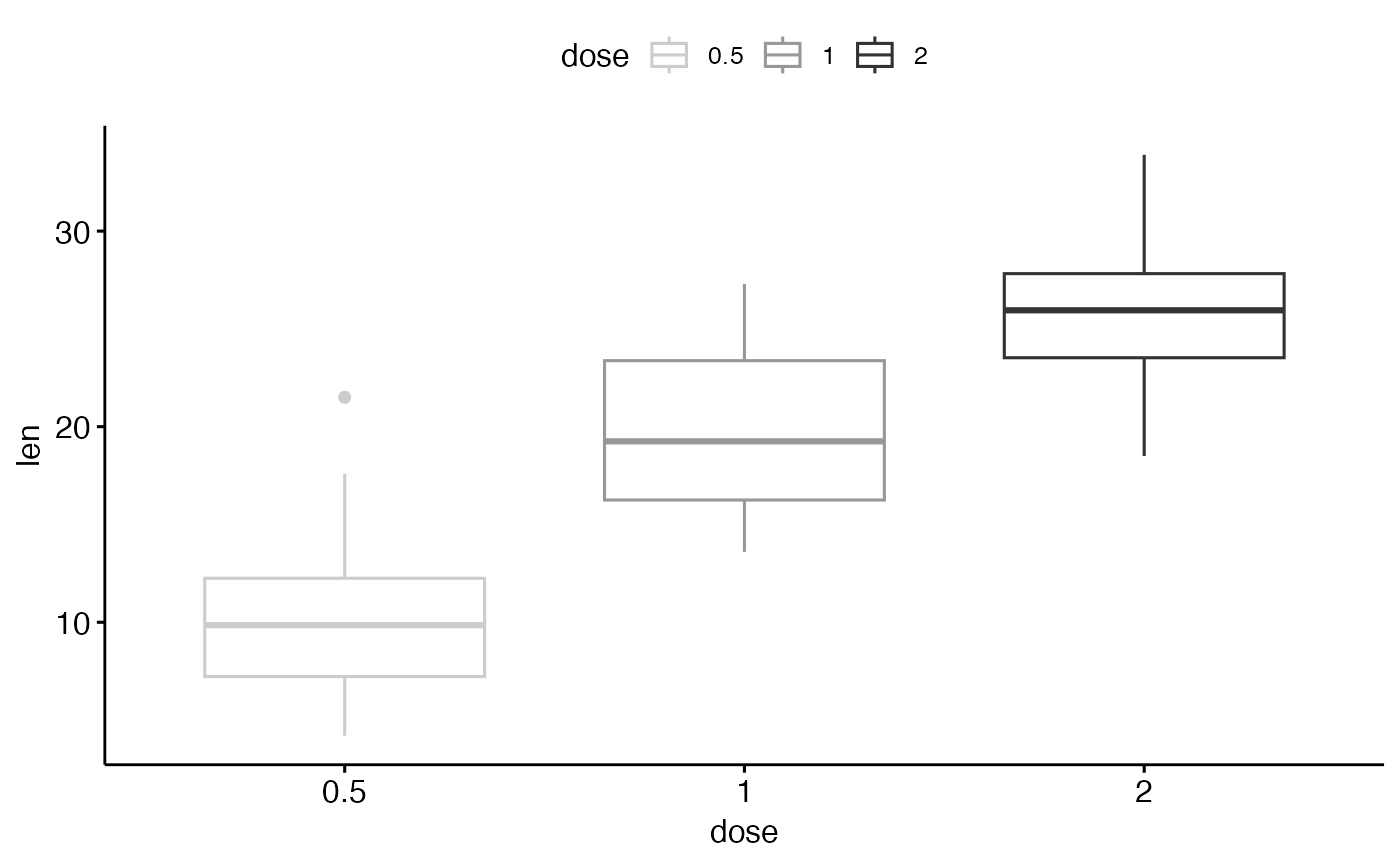# Use scientific journal palette from ggsci package
ggpar(p2, palette = "npg") # nature# Axis ticks, limits, scales
# +++++++++++++++++++++++++

# Axis ticks labels and rotation
ggpar(p,
font.tickslab = c(14,"bold", "#993333"),
xtickslab.rt = 45, ytickslab.rt = 45)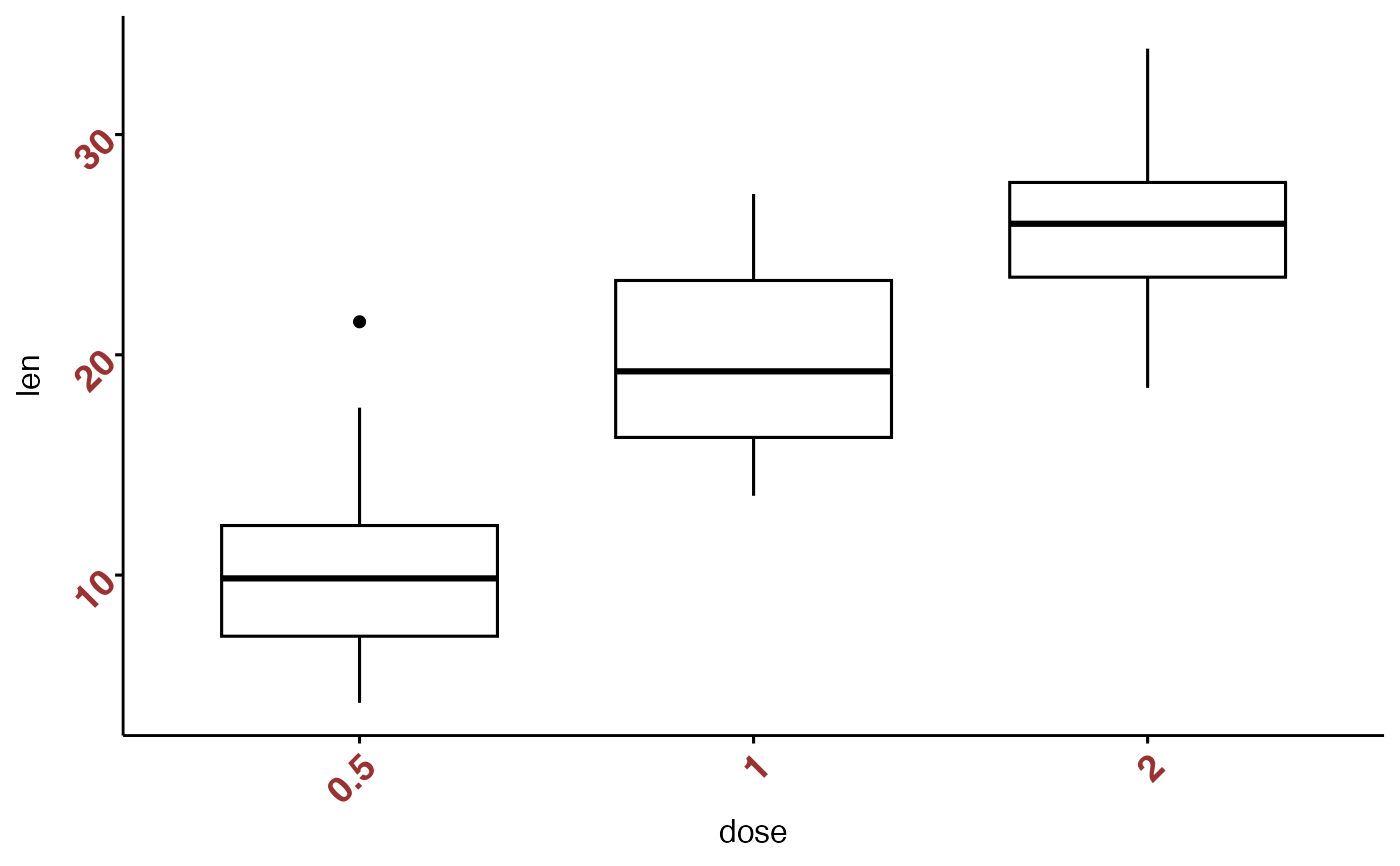# Hide axis ticks and tick labels
ggpar(p, ticks = FALSE, tickslab = FALSE)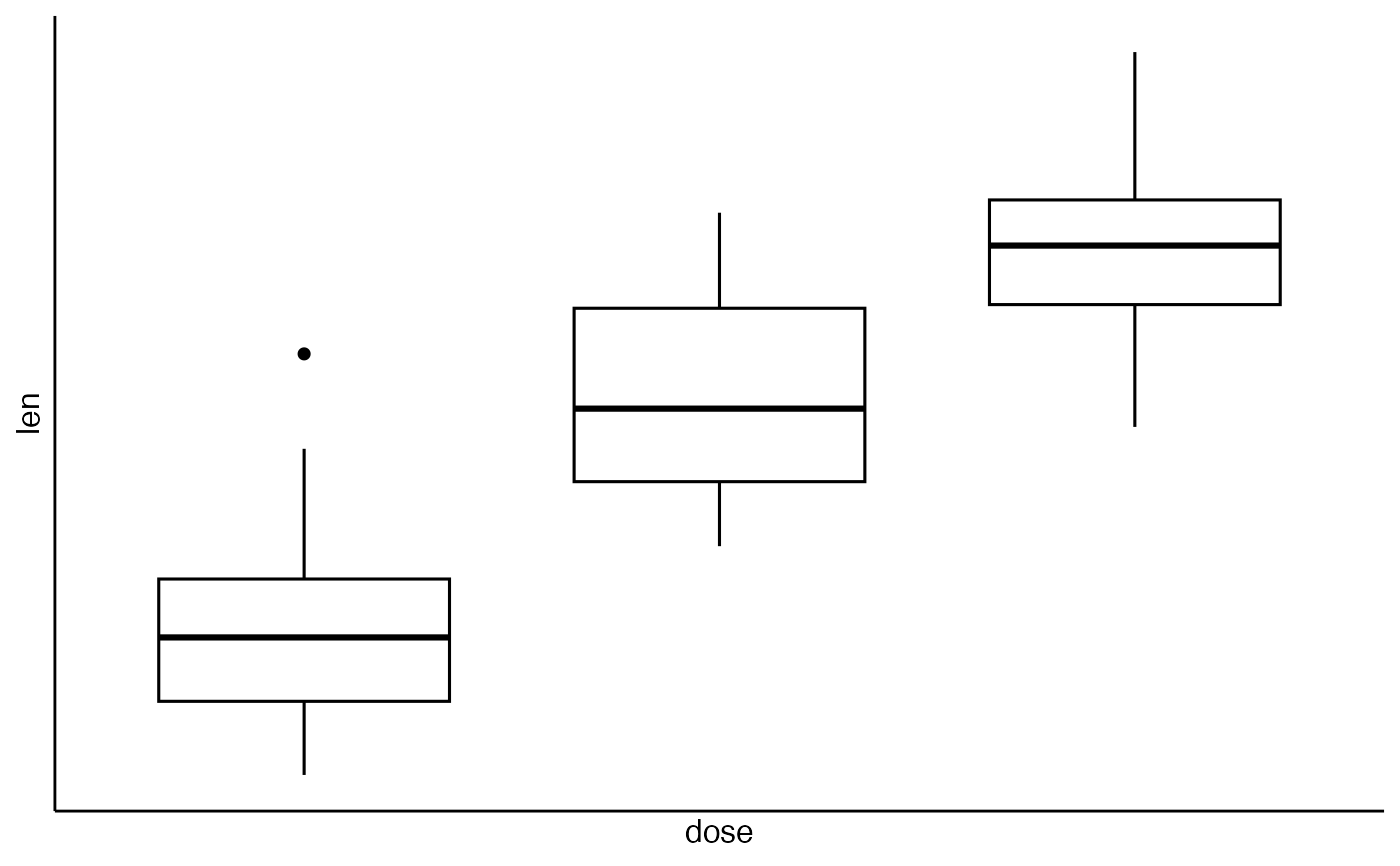# Axis limits
ggpar(p, ylim = c(0, 50))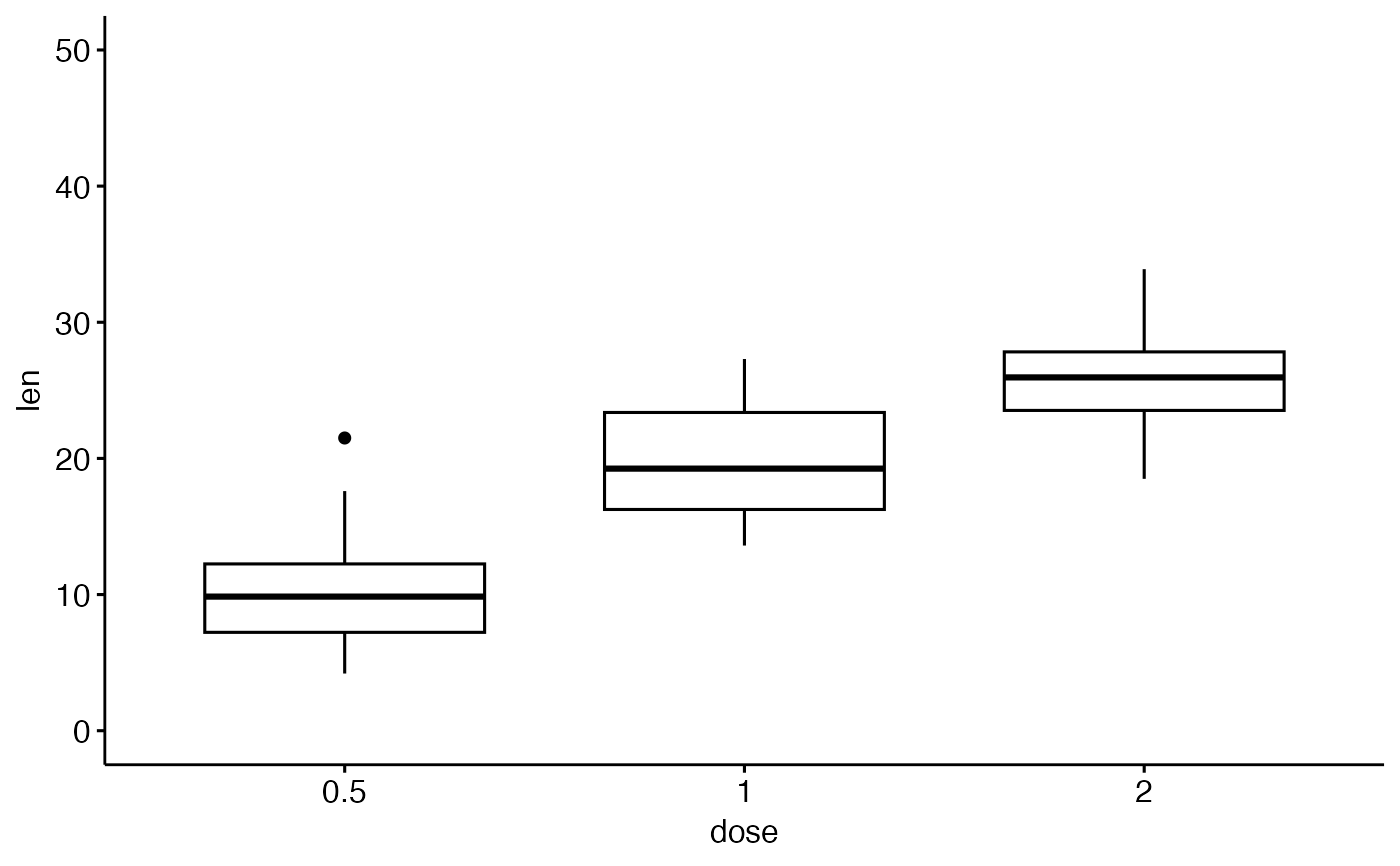# Axis scale
ggpar(p, yscale = "log2")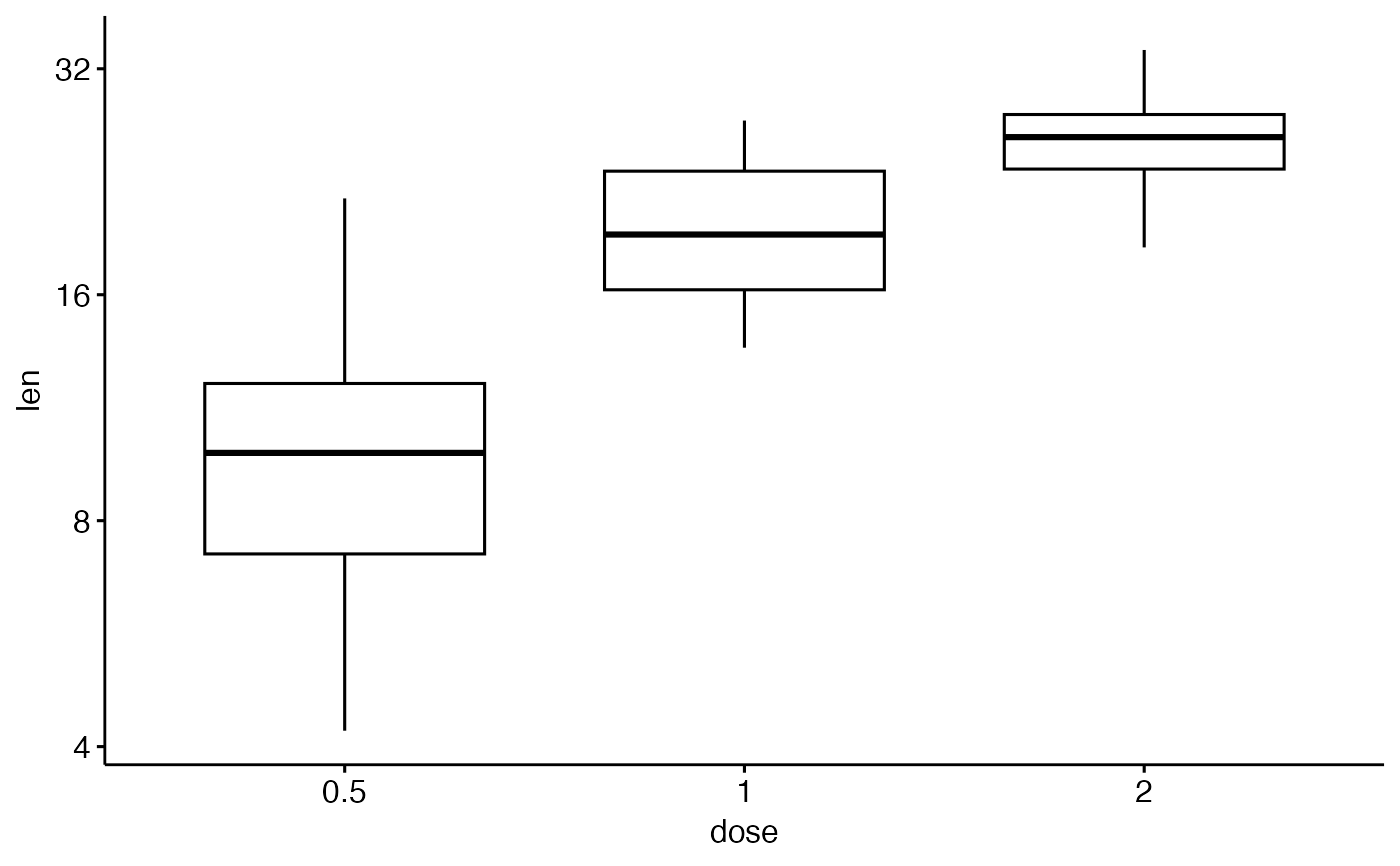# Format axis scale
ggpar(p, yscale = "log2", format.scale = TRUE)# Legends
# ++++++++++++++++++
# Change legend position and title
ggpar(p2,
legend = "right", legend.title = "Dose (mg)",
font.legend = c(10, "bold", "red"))